# RSoft & Photonic Solutions Enewsletter

## Case Study: Modeling Probabilistic Constellation Shaping Using OptSim's Digital Signal Processing Library

Modern long-haul fiber-optic transmission systems use polarization multiplexing with advanced modulation formats to transport large amounts of data with higher spectral efficiency, and coherent reception with digital signal processing (DSP) to recover the transmitted information. This article discusses modeling of probabilistically shaped (PS) coherent systems in OptSim, a topic that has attracted a lot of attention lately in order to achieve the data transmission capacity close to the Shannon limit.

Basics of Probabilistic Shaping

Probabilistic Constellation Shaping (PCS) is a DSP technique that transmits a quadrature amplitude modulated (QAM) signal, where each constellation point is transmitted with a different probability . In general, this technique provides two advantages over standard QAM transmission:

1. High data rate flexibility (changing the probability to be transmitted of a constellation reduces, in general, its data rate).
2. Small sensitivity gain, due to a better “match” between constellation and channel.

For these two reasons, PCS has been widely adopted in optical communications.

In general, the probability distribution of constellation points can be arbitrarily chosen. In practice, the optical channel is very well approximated by an additive white Gaussian noise (AWGN) channel, i.e., a channel that adds a random white Gaussian noise. For this channel, it has been shown that the optimal distribution is the Maxwell-Boltzmann distribution: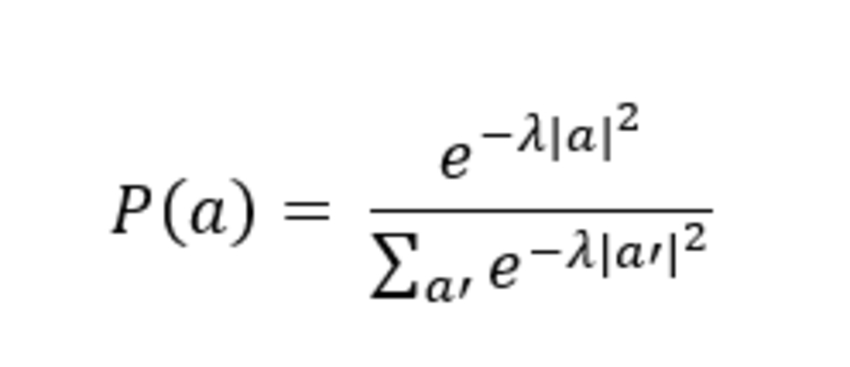where ɑ is the complex-valued constellation point. The parameter λ tunes the amount of shaping that is applied to the constellation. A value of λ = 0 corresponds to an unshaped (standard) constellation, while for the increase of λ the outer points are transmitted with lesser probability. This operation reduces the data rate and at the same time slightly increases the receiver sensitivity.

In a realistic PCS transmitter, the match between a random stream of bits to a set of amplitudes with a given distribution is performed by an algorithm called Distribution Matcher (DM), and its inverse operation at the receiver is performed by an Inverse Distribution Matcher (IDM). To avoid error bursts, the IDM requires error-free sequences. Therefore, Forward Error Correction (FEC) needs to be applied after PS in a way that does not change symbol probabilities. A scheme to perform this operation is the Probability Amplitude Shaping (PAS) algorithm. With this scheme, the net (i.e., post-FEC) data rate is equal to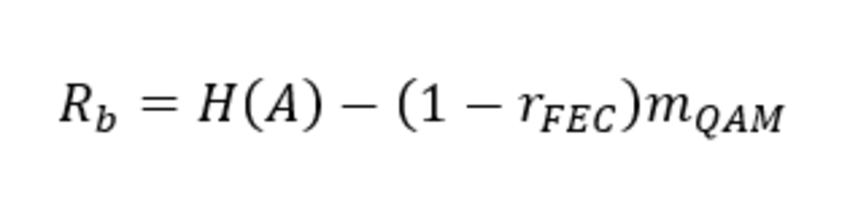In this equation, H (A) = -ΣP (ɑ) log2 P(ɑ) is the entropy of the transmit constellation, rFEC is the rate of the FEC code, and mQAM  is the number of bit/symbol of the QAM constellation. The entropy of the constellation is linked to the parameterλ: increasing  λ, the entropy (and, therefore, the data rate) is reduced.

Figure 1 depicts the entropy of a probabilistically shaped (PS)-64-QAM constellation for different values of  λ.

For  λ = 0, the constellation is unshaped, and the entropy is equal to the base-2 logarithm of the number of points. Increasing  λ, the entropy reduces.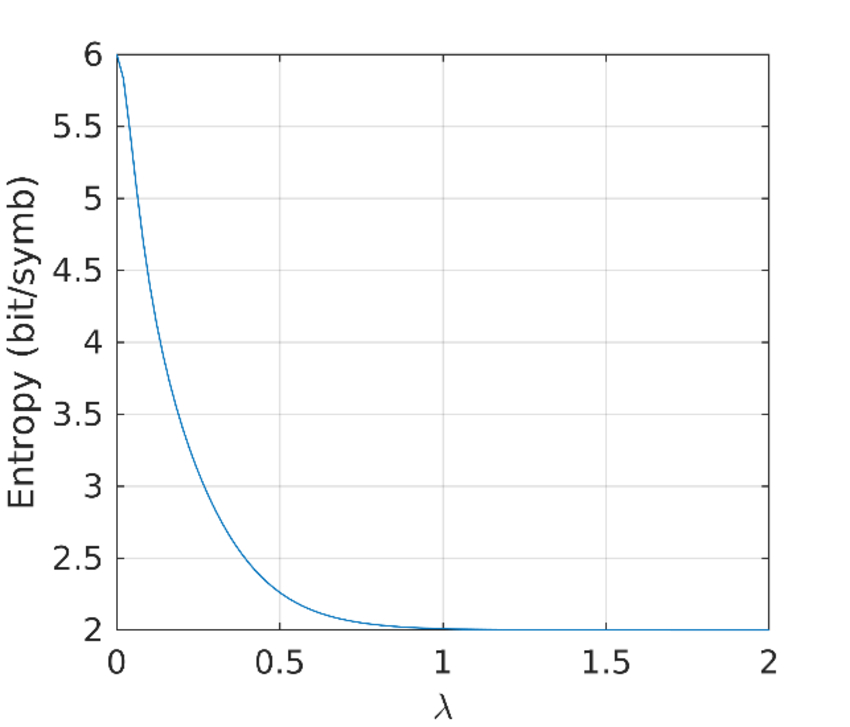Figure 1: Entropy of a PS-64-QAM constellation

At the receiver, the performance of a PCS transmission can be evaluated using an appropriate performance metric without performing an actual FEC decoding, which would require an overly large number of bits to perform reliable post-FEC BER measurements. In literature [2-3], the most-used performance metric is the Generalized Mutual Information (GMI). For large values of SNR, the GMI saturates to the entropy of the constellation. Reducing the SNR also reduces the GMI.

OptSim Simulation Setup and Results

Figure 2 shows the schematic setup of a PS-64-QAM system in OptSim. The transmitter transmits a square-QAM signal (e.g., 64-QAM), where the points are transmitted according to a Maxwell-Boltzmann distribution.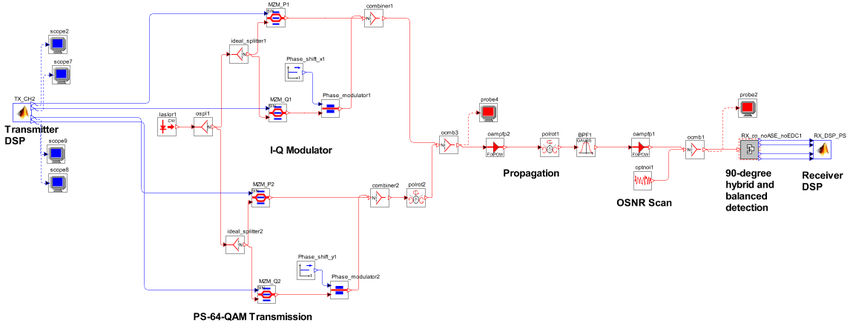Figure 2: Schematic of the OptSim Simulation Setup for PS-64-QAM Transmission

The PCS signal is tuned with two parameters: (i) the Maxwell-Boltzmann  parameter; and (ii) the seed of the random PS QAM sequence generator.

Figures 3 and 4 show results obtained with this example. Figure 3 shows the GMI of a standard 64-QAM, which is compared to PS-64-QAM with λ = 0.1, which corresponds to an entropy H(A) = 4.41 bit/symbol. This means that the data rate of PS-64-QAM is lower than 64-QAM. Assuming an ideal 20%-overhead FEC, 64-QAM would transmit at a 320 Gbit/s net data rate, while PS-64-QAM would transmit at a 218.24 Gbit/s data rate.

As seen in Figure 3, each GMI converges, for large values of SNRs, to the constellation entropy. However, for small values of OSNR, PS-64-QAM has a slightly higher GMI than 64-QAM, which means that it is more efficient than   64-QAM at a fixed OSNR. A scatter plot of the PS-64-QAM is shown in Figure 4.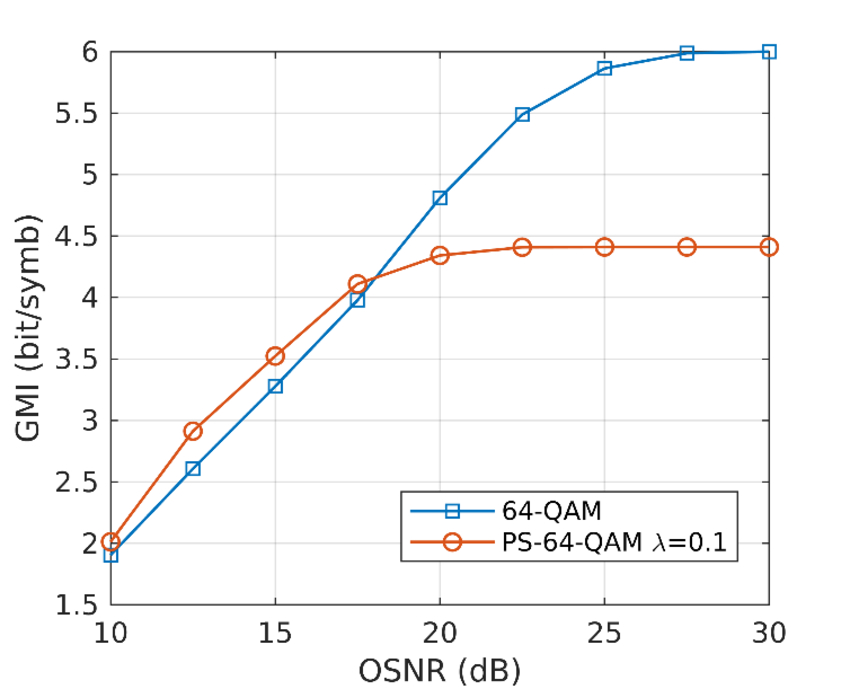Figure 3: Comparison of 64-QAM with PS-64-QAM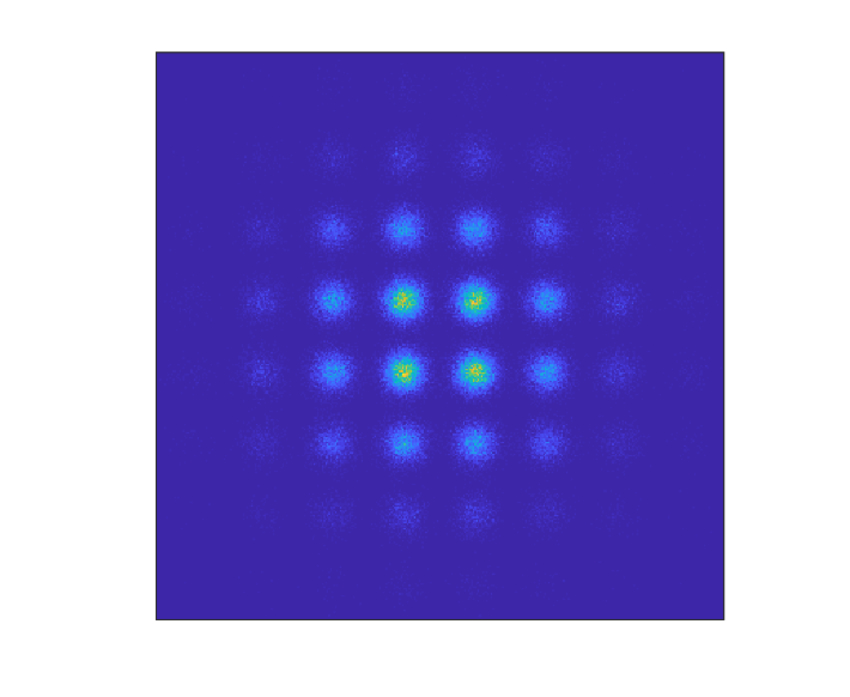Figure 4: Scatter plot of PS-64-QAM

##### References
1. Junho Cho and Peter J. Winzer, “Probabilistic Constellation Shaping for Optical Fiber Communications”, J. Lightwave Technol. (2019).
2. Dario Pilori, Luca Bertignono, Antonino Nespola, Fabrizio Forghieri, and Gabriella Bosco, "Comparison of Probabilistically Shaped 64QAM With Lower Cardinality Uniform Constellations in Long-Haul Optical Systems," J. Lightwave Technol. 36, 501-509 (2018).
3. Fred Buchali, Fabian Steiner, Georg Böcherer, Laurent Schmalen, Patrick Schulte, and Wilfried Idler, "Rate Adaptation and Reach Increase by Probabilistically Shaped 64-QAM: An Experimental Demonstration," J. Lightwave Technol. 34, 1599-1609 (2016).

## University of Rochester PIC TrainingMay 14-17, 2019
University of Rochester
Rochester, NY

University of Rochester Medical Center, and Synopsys present a four-day training on photonic design for photonic integrated circuits (PICs). The training is aimed at academic and corporate parties interested in understanding the development of PICs and the role of software automation in their design, simulation, and fabrication procedures.

During this training, participants will receive an overview of the integrated photonics eco-system, together with an introduction to Synopsys PIC Design Tools and their role in integrated photonics design and manufacturing.

This training will feature live demonstrations and hands-on session using Synopsys PIC Design Tools for PDK-driven PIC design. During the hands-on session, attendees will have the opportunity to work directly with the software under the guidance of expert technical staff from Synopsys.

## Calendar of Events

For the latest event calendar, please go to our Events page.